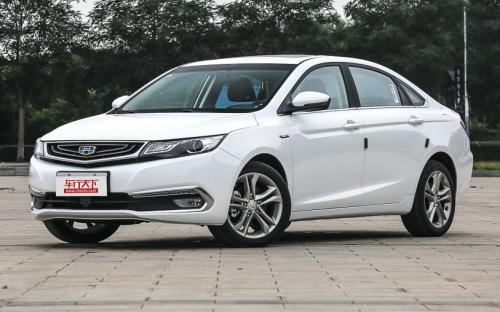吉利汽车 帝豪GL0 种颜色可选2017款最低售价：7.88 万元起

4725(mm)1802(mm)1478(mm)配置亮点：
• 胎压监测装置

• ISOFIX儿童座椅接口

• 车身稳定控制(ESC/ESP/DSC等)

• 电动天窗

• 定速巡航

• 后倒车雷达

• 真皮座椅

• GPS导航系统

• 氙气大灯

• 后视镜加热

• 提交
2017款 1.3T 自动精英型 (119张)
• 2017款 1.3T 自动精英型 (119张)
• 2017款 1.3T 自动尊贵型 (100张)
• 2017款 1.8L 自动精英型 (99张)
• 吉利汽车 帝豪GL 绕车实拍• 吉利汽车 帝豪GL 在售车型

排量 车型 厂商指导价 本地最低报价 购车工具
1.8L
7.88万
8.58万
1.8L 自动精英型 6挡双离合
9.58万
1.3T
8.78万
1.3T 自动精英型 6挡双离合
9.78万
1.3T 自动尊贵型 6挡双离合
10.78万
1.3T 自动旗舰型 6挡双离合
11.38万

吉利汽车 帝豪GL 经销商

查看更多 >>

吉利汽车 帝豪GL 动力加速

帝豪GL 0-100公里加速时间分布在 0.0-秒 属于 超跑级

动力级别 加速时间 车型

吉利汽车 帝豪GL 视频

吉利汽车 帝豪GL 新闻资讯

谁是一哥 自主紧凑轿车艾瑞泽5深度PK帝豪GL

新闻 超过12917次关注

艾瑞泽5和帝豪GL二者价格定位相仿、配置相近，且有着十分相似的目标群体，人们对于这两款车的比较一直未停歇。今天我们不妨换个视角，将对比维度进行延伸和拓展，看...

以自胜之道向上突破 吉利帝豪GL实力体验营郑州站12月11日全面开启

本地资讯 超过8281次关注

吉利帝豪GL实力体验营—郑州站倒计时2天！12月11日黄帝宫御温泉邀您见证强者实力！河南华泽体验官招募中！报名热线：0371-55092555吉利帝豪GL实力体验营——比肩合...

吉利帝豪GL正式上市 售价7.88-11.38万

上市新车 超过11353次关注

2016年9月20日，吉利宣布帝豪GL正式上市，新车分别搭载1.3T、1.8L两款发动机，共分7款车型，售价区间为7.88-11.38万元。帝豪GL基于吉利FE平台打造，轴距为2700mm，定位为A+级车型。

9月20日将上市 吉利帝豪GL实车到店

国产新车 超过8583次关注

近日获悉，吉利帝豪GL已经陆续到店，同时我们也在北京某经销商拍摄到1.3T顶配车型的商品车。根据之前消息，新车将在9月20日上市，并将提供1.3T和1.8L两款发动机选择。

美国驻华大使为博越点赞 吉利汽车成G20峰会中国品牌代言人

行业动态 超过10518次关注

9月4日-5日，二十国集团领导人第十一次峰会在杭州国际博览中心举行（以下简称“杭州G20峰会”）。作为全球最具影响力的政治经济论坛，杭州G20峰会汇聚了全球的目光...

猜你喜欢

﻿
• 快速找车
• 选择品牌
• 选择品牌
• A  奥迪
• A  阿斯顿·马丁
• A  阿尔法·罗密欧
• B  宝沃
• B  布加迪
• B  巴博斯
• B  保时捷
• B  宾利
• B  奔驰
• B  宝马
• B  本田
• B  别克
• B  标致
• B  比亚迪
• B  宝骏
• B  北汽制造
• B  北汽新能源
• B  北汽幻速
• B  北汽威旺
• B  北京汽车
• B  奔腾
• B  北汽绅宝
• C  长安
• C  长安商用
• C  长城
• C  昌河
• D  大众
• D  道奇
• D  DS
• D  东南
• D  东风风神
• D  东风风行
• D  东风小康
• D  东风风度
• D  东风
• F  福特
• F  丰田
• F  菲亚特
• F  法拉利
• F  福田
• F  福迪
• F  福汽启腾
• G  观致
• G  广汽传祺
• G  广汽吉奥
• G  GMC
• H  红旗
• H  汉腾汽车
• H  哈弗
• H  哈飞
• H  海格
• H  海马
• H  华颂
• H  黄海
• H  华泰
• H  恒天
• J  吉利汽车
• J  捷豹
• J  Jeep
• J  江淮
• J  江铃
• J  金杯
• J  九龙
• J  金旅
• K  凯翼
• K  凯迪拉克
• K  克莱斯勒
• K  科尼塞克
• K  卡威
• K  开瑞
• L  路虎
• L  林肯
• L  劳斯莱斯
• L  兰博基尼
• L  雷克萨斯
• L  铃木
• L  雷诺
• L  理念
• L  力帆
• L  莲花汽车
• L  猎豹
• L  路特斯
• L  陆风
• M  马自达
• M  MG
• M  MINI
• M  玛莎拉蒂
• M  摩根
• M  迈凯轮
• N  纳智捷
• O  欧宝
• O  讴歌
• O  欧朗
• Q  奇瑞
• Q  起亚
• Q  启辰
• R  日产
• R  荣威
• R  瑞麒
• S  三菱
• S  斯威汽车
• S  萨博
• S  smart
• S  斯柯达
• S  斯巴鲁
• S  思铭
• S  双龙
• S  上汽大通
• S  双环
• T  特斯拉
• T  腾势
• W  沃尔沃
• W  五菱汽车
• W  五十铃
• W  威兹曼
• W  威麟
• X  现代
• X  雪佛兰
• X  雪铁龙
• X  西雅特
• Y  一汽
• Y  英菲尼迪
• Y  英致
• Y  依维柯
• Y  野马汽车
• Y  永源
• Z  众泰
• Z  中华
• Z  中兴
• Z  知豆
• 选择车系
• 选择车系
• 车型对比
• 选择品牌
• 选择品牌
• A  奥迪
• A  阿斯顿·马丁
• A  阿尔法·罗密欧
• B  宝沃
• B  布加迪
• B  巴博斯
• B  保时捷
• B  宾利
• B  奔驰
• B  宝马
• B  本田
• B  别克
• B  标致
• B  比亚迪
• B  宝骏
• B  北汽制造
• B  北汽新能源
• B  北汽幻速
• B  北汽威旺
• B  北京汽车
• B  奔腾
• B  北汽绅宝
• C  长安
• C  长安商用
• C  长城
• C  昌河
• D  大众
• D  道奇
• D  DS
• D  东南
• D  东风风神
• D  东风风行
• D  东风小康
• D  东风风度
• D  东风
• F  福特
• F  丰田
• F  菲亚特
• F  法拉利
• F  福田
• F  福迪
• F  福汽启腾
• G  观致
• G  广汽传祺
• G  广汽吉奥
• G  GMC
• H  红旗
• H  汉腾汽车
• H  哈弗
• H  哈飞
• H  海格
• H  海马
• H  华颂
• H  黄海
• H  华泰
• H  恒天
• J  吉利汽车
• J  捷豹
• J  Jeep
• J  江淮
• J  江铃
• J  金杯
• J  九龙
• J  金旅
• K  凯翼
• K  凯迪拉克
• K  克莱斯勒
• K  科尼塞克
• K  卡威
• K  开瑞
• L  路虎
• L  林肯
• L  劳斯莱斯
• L  兰博基尼
• L  雷克萨斯
• L  铃木
• L  雷诺
• L  理念
• L  力帆
• L  莲花汽车
• L  猎豹
• L  路特斯
• L  陆风
• M  马自达
• M  MG
• M  MINI
• M  玛莎拉蒂
• M  摩根
• M  迈凯轮
• N  纳智捷
• O  欧宝
• O  讴歌
• O  欧朗
• Q  奇瑞
• Q  起亚
• Q  启辰
• R  日产
• R  荣威
• R  瑞麒
• S  三菱
• S  斯威汽车
• S  萨博
• S  smart
• S  斯柯达
• S  斯巴鲁
• S  思铭
• S  双龙
• S  上汽大通
• S  双环
• T  特斯拉
• T  腾势
• W  沃尔沃
• W  五菱汽车
• W  五十铃
• W  威兹曼
• W  威麟
• X  现代
• X  雪佛兰
• X  雪铁龙
• X  西雅特
• Y  一汽
• Y  英菲尼迪
• Y  英致
• Y  依维柯
• Y  野马汽车
• Y  永源
• Z  众泰
• Z  中华
• Z  中兴
• Z  知豆
• 选择车系
• 选择车系
• 选择车型
• 选择车型
• 意见反馈